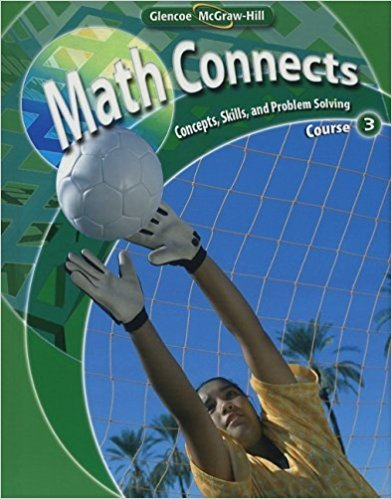×
×

# Solutions for Chapter 2: Math Connects: Concepts, Skills, and Problem Solving Course 3 0th Edition## Full solutions for Math Connects: Concepts, Skills, and Problem Solving Course 3 | 0th Edition

ISBN: 9780078740503Solutions for Chapter 2

Solutions for Chapter 2
4 5 0 414 Reviews
12
0
##### ISBN: 9780078740503

This textbook survival guide was created for the textbook: Math Connects: Concepts, Skills, and Problem Solving Course 3, edition: 0. This expansive textbook survival guide covers the following chapters and their solutions. Since 12 problems in chapter 2 have been answered, more than 1174 students have viewed full step-by-step solutions from this chapter. Chapter 2 includes 12 full step-by-step solutions. Math Connects: Concepts, Skills, and Problem Solving Course 3 was written by and is associated to the ISBN: 9780078740503.

Key Math Terms and definitions covered in this textbook
• Back substitution.

Upper triangular systems are solved in reverse order Xn to Xl.

• Basis for V.

Independent vectors VI, ... , v d whose linear combinations give each vector in V as v = CIVI + ... + CdVd. V has many bases, each basis gives unique c's. A vector space has many bases!

• Cyclic shift

S. Permutation with S21 = 1, S32 = 1, ... , finally SIn = 1. Its eigenvalues are the nth roots e2lrik/n of 1; eigenvectors are columns of the Fourier matrix F.

• Fibonacci numbers

0,1,1,2,3,5, ... satisfy Fn = Fn-l + Fn- 2 = (A7 -A~)I()q -A2). Growth rate Al = (1 + .J5) 12 is the largest eigenvalue of the Fibonacci matrix [ } A].

• Graph G.

Set of n nodes connected pairwise by m edges. A complete graph has all n(n - 1)/2 edges between nodes. A tree has only n - 1 edges and no closed loops.

• Kirchhoff's Laws.

Current Law: net current (in minus out) is zero at each node. Voltage Law: Potential differences (voltage drops) add to zero around any closed loop.

• Left inverse A+.

If A has full column rank n, then A+ = (AT A)-I AT has A+ A = In.

• Linear transformation T.

Each vector V in the input space transforms to T (v) in the output space, and linearity requires T(cv + dw) = c T(v) + d T(w). Examples: Matrix multiplication A v, differentiation and integration in function space.

• Lucas numbers

Ln = 2,J, 3, 4, ... satisfy Ln = L n- l +Ln- 2 = A1 +A~, with AI, A2 = (1 ± -/5)/2 from the Fibonacci matrix U~]' Compare Lo = 2 with Fo = O.

• Matrix multiplication AB.

The i, j entry of AB is (row i of A)·(column j of B) = L aikbkj. By columns: Column j of AB = A times column j of B. By rows: row i of A multiplies B. Columns times rows: AB = sum of (column k)(row k). All these equivalent definitions come from the rule that A B times x equals A times B x .

• Multiplication Ax

= Xl (column 1) + ... + xn(column n) = combination of columns.

• Nilpotent matrix N.

Some power of N is the zero matrix, N k = o. The only eigenvalue is A = 0 (repeated n times). Examples: triangular matrices with zero diagonal.

• Normal matrix.

If N NT = NT N, then N has orthonormal (complex) eigenvectors.

• Orthonormal vectors q 1 , ... , q n·

Dot products are q T q j = 0 if i =1= j and q T q i = 1. The matrix Q with these orthonormal columns has Q T Q = I. If m = n then Q T = Q -1 and q 1 ' ... , q n is an orthonormal basis for Rn : every v = L (v T q j )q j •

• Pseudoinverse A+ (Moore-Penrose inverse).

The n by m matrix that "inverts" A from column space back to row space, with N(A+) = N(AT). A+ A and AA+ are the projection matrices onto the row space and column space. Rank(A +) = rank(A).

• Row picture of Ax = b.

Each equation gives a plane in Rn; the planes intersect at x.

• Schwarz inequality

Iv·wl < IIvll IIwll.Then IvTAwl2 < (vT Av)(wT Aw) for pos def A.

• Singular matrix A.

A square matrix that has no inverse: det(A) = o.

• Special solutions to As = O.

One free variable is Si = 1, other free variables = o.

• Trace of A

= sum of diagonal entries = sum of eigenvalues of A. Tr AB = Tr BA.

×